## 2.6. The peculiar nature of and and or

In Python, and and or perform boolean logic as you would expect, but they do not return boolean values; they return one of the actual values they are comparing.

### Example 2.16. Introducing and

```>>> 'a' and 'b'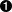'b'
>>> '' and 'b'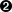''
>>> 'a' and 'b' and 'c'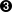'c'```When using and, values are evaluated in a boolean context from left to right. 0, '', [], (), {}, and None are false in a boolean context; everything else is true. If all values are true in a boolean context, and returns the last value. In this case, and evaluates 'a', which is true, then 'b', which is true, and returns 'b'.If any value is false in a boolean context, and returns the first false value. In this case, '' is the first false value.All values are true, so and returns the last value, 'c'.

### Example 2.17. Introducing or

```>>> 'a' or 'b''a'
>>> '' or 'b''b'
>>> '' or [] or {}{}
>>> def sidefx():
...     print "in sidefx()"
...     return 1
>>> 'a' or sidefx()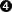'a'```When using or, values are evaluated in a boolean context from left to right, just like and. If any value is true, or returns that value immediately. In this case, 'a' is the first true value.or evaluates '', which is false, then 'b', which is true, and returns 'b'.If all values are false, or returns the last value. or evaluates '', which is false, then [], which is false, then {}, which is false, and returns {}.Note that or only evaluates values until it finds one that is true in a boolean context, and then it ignores the rest. This distinction is important if some values can have side effects. Here, the function sidefx is never called, because or evaluates 'a', which is true, and returns 'a' immediately.

If you’re a C hacker, you are certainly familiar with the bool ? a : b expression, which evaluates to a if bool is true, and b otherwise. Because of the way and and or work in Python, you can accomplish the same thing.

### Example 2.18. Introducing the and-or trick

```>>> a = "first"
>>> b = "second"
>>> 1 and a or b'first'
>>> 0 and a or b'second'
```This syntax looks similar to the bool ? a : b expression in C. The entire expression is evaluated from left to right, so the and is evaluated first. 1 and 'first' evalutes to 'first', then 'first' or 'second' evalutes to 'first'.0 and 'first' evalutes to 0, then 0 or 'second' evaluates to ’second'.

However, since this Python expression is simply boolean logic, and not a special construct of the language, there is one very, very, very important difference between this and-or trick in Python and the bool ? a : b syntax in C. If the value of a is false, the expression will not work as you would expect it to. (Can you tell I was bitten by this? More than once?)

### Example 2.19. When the and-or trick fails

```>>> a = ""
>>> b = "second"
>>> 1 and a or b'second'```Since a is an empty string, which Python considers false in a boolean context, 1 and '' evalutes to '', then '' or 'second' evalutes to 'second'. Oops! That’s not what we wanted.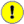The and-or trick, bool and a or b, will not work like the C expression bool ? a : b when a is false in a boolean context.

The real trick behind the and-or trick, then, is to make sure that the value of a is never false. One common way of doing this is to turn a into [a] and b into [b], then taking the first element of the returned list, which will be either a or b.

### Example 2.20. Using the and-or trick safely

```>>> a = ""
>>> b = "second"
>>> (1 and [a] or [b])''```Since [a] is a non-empty list, it is never false. Even if a is 0 or '' or some other false value, the list [a] is true because it has one element.

By now, this trick may seem like more trouble than it’s worth. You could, after all, accomplish the same thing with an if statement, so why go through all this fuss? Well, in many cases, you are choosing between two constant values, so you can use the simpler syntax and not worry, because you know that the a value will always be true. And even if you have to use the more complicated safe form, there are good reasons to do so; there are some cases in Python where if statements are not allowed, like lambda functions.